# [FREE] Maths Extension

1. Introduction
Use this extension to do simple and Advanced Mathematics.

2. Details
Name: Maths
Version: `1`
Package Name: `com.appybuilder.rutheniumalpha.Maths`
Date Build: 2021-02-06T18:30:00Z
Last Updated: 2021-02-06T18:30:00Z

3. Blocks

Absolute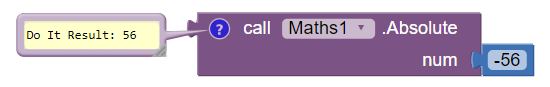Returns the absolute value of num.

AngleThetaRectanCoord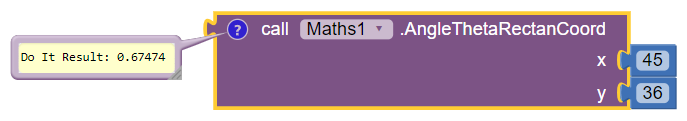Returns the angle theta from the conversion of rectangular coordinates (x, y) to polar coordinates.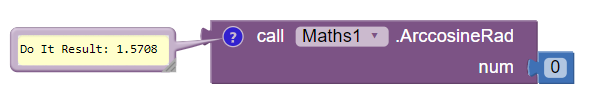Returns the arccosine of num, in radians.Returns the arcsine of num, in radians.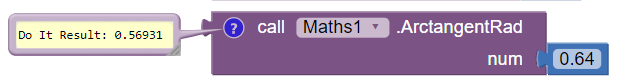Returns the arctangent of num as a numeric value between -PI/2 and PI/2 radians.

Arithemetics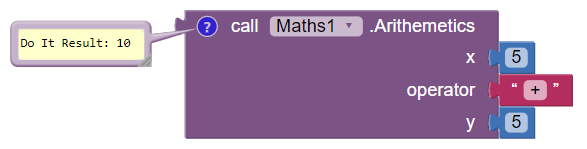Use Arithemetics. + for Adddition, - for Subtraction, * for Multiplication, / for Division.

Ceil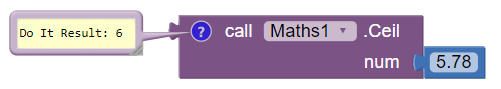Returns the value of num rounded up to its nearest integer.

Convert
Converts Radians to Degrees and vise versa. Rad - Radians, Deg - Degrees. If the from-to is not given properly it will return 0.
Case 1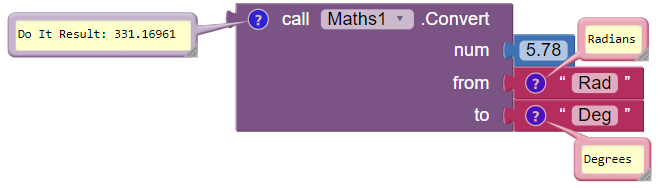This will convert Radians to Degrees.
Case 2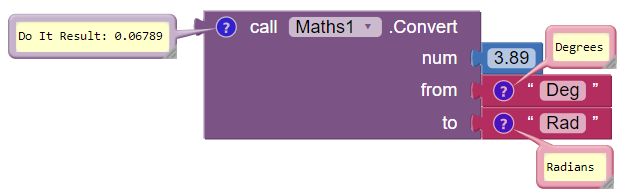This will convert Degrees to Radians.

Cosine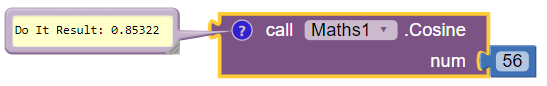Returns the cosine of num (num is in radians).

CubeRoot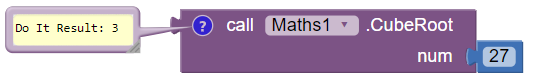Returns the cube root of num.

FloorReturns the value of num rounded down to its nearest integer.

HyperbolicCosine and HyperbolicTangent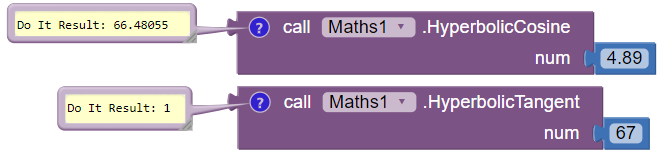Returns the Hyperbolic Tangent/Cosine of num.

Hypot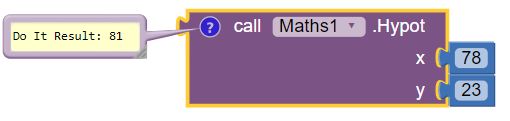Returns sqrt(x^2 +y^2) without intermediate overflow or underflow.

Log and Log10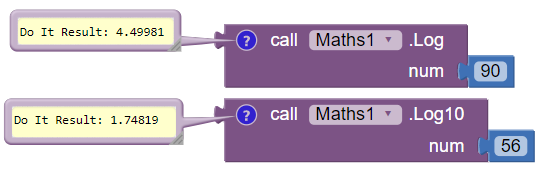Returns the log/log 10 of num.

Min and Max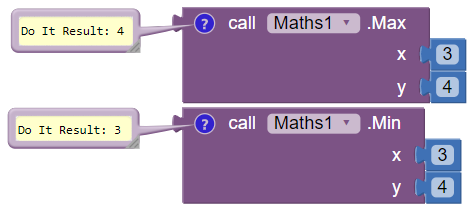Returns the min/max of x,y.

Power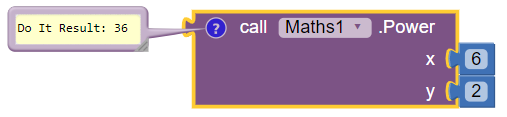Returns the value of x to the power of y.

Random0to1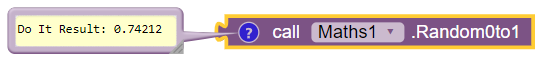Returns a random number between 0 and 1.

Round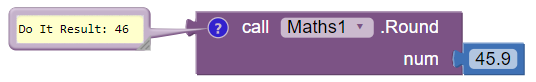Returns the value of num rounded to its nearest integer.

Sine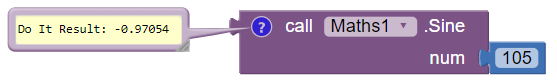Returns the sine of num (num is in radians).

SquareRootReturns the square root of num.

Tangent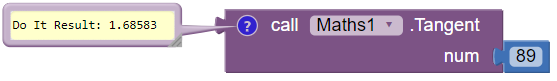Returns the tangent of an angle.

CopySign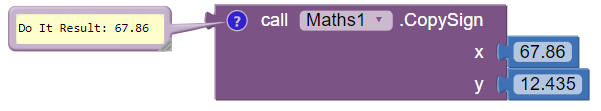Returns the first floating point num with the sign of the second floating point y.

Thanks to my best friend and teacher @Salman_Dev.Please feel free to say any improvements or any bugs.7 Likes

Maybe add a function called Map()? I know it can be achieved by regular blocks though.

4 Likes

Nice extension @Alpha2020

2 Likes

Thank you very much @Nisarga_Adhikary2 Likes

Wow, I’m so proud of you. @Alpha2020Keep up the good work.3 Likes

Thank you....3 Likes

Some blocks are useful,
others are present in the inbuilt blocks itself...

4 Likes

Good. Can you adjust the size of the image of the extension? I think it is too big.